﻿ H.G. de Wolff - The IS-ML model

## The IS-LM model

### First insertion on Heterodox Gazette Sam de Wolff: 21 july 2014

E.A. Bakkum is a blogger for the Sociaal Consultatiekantoor. He loves to reflect on the labour movement.

This column presents the well-known IS/LM model, that tries to explain the interaction between the commodity market and the money market. The commodity market is dominated by the investment function. On the money market especially the speculation is relevant. The two markets affect each other by means of the rate of interest. Some believe that the IS/LM model is a synthesis of the neoclassical paradigm and the theory of Keynes, but this opinion does not do justice to the large differences between both paradigms.

The following text is an almost literal copy of the paragraph 12.7 in the provisional reader Vooruitgang der economische wetenschap, which your columnist issued in 2011 with the publisher E. de Bibelude, a part of the Sociaal Consultatiekantoor. The reader is meant to be an introduction, and that is certainly true for this monetary part, which is superficial in substance - and thus hopefully easy to read. In the preceding column the fundamental concepts with regard to money and capital have already been defined. In a future column the IS/LM model will be refined further.

### A neoclassical interpretation of de Kaleckian-Keynesian theory

In the Kaleckian-Keynesian theory the investments are essential in order to keep the national income Y at the desired level. The producers and the state pay the investments mostly by means of loans, and must pay an interest as a compensation for the use of the capital. It is commonly assumed, that the height of the rate of interest rk is hardly important for the decision to borrow capital. The banks are usually willing to create the money, that is required for the expansion, without adapting the rate of interest. This idea is a hallmark of the Kaleckian-Keynesian theory, and leads to a controversy with the neoclassical theory and with the monetarists.

For, in the neoclassical theory the interest is simply the price of the money capital, and the producers will weigh its height just like they do for any other costs in the production process. Reversely, the propensity to save of the households will always increase, as soon as the rate of interest is raised. In a previous column it has already been remarked, that the economist Keynes was formed in the neoclassical tradition, and therefore attached some meaning to the interest in the formulation of his theory. This distinguishes him from his congenials in the economics of dynamic processes, such as Kalecki and the post-keynesians.

The rupture of Keynes with the monetarism is expressed by his denial of the necessity of the equilibrium S=I between the savings and the investments (at least with regard to the short term). The economists J.R. Hicks and A.H. Hansen have built on the interest-model of Keynes, and developed it into the IS/LM model. It illustrates how the themes concerning money and capital, which have been discussed in the previous column, can be integrated in the economic theory. Therefore the IS/LM model is sometimes called the synthesis of the Kaleckian-Keynesian theory and monetarism. Since the IS/LM model has been developed for the description of short-term phenomena, the prices are kept constant in all derivations.

### The sphere of commodities

The IS/LM model actually consists of two parts, namely the IS part with regard to the real economy of the commodity production, and the LM part with regard to the monetary policy. The rate of interest connects the commodity sphere of the IS part with the money sphere of the LM part, and brings about the stability of the economy. In this column the parts are first discussed separately1. For the sake of convenience, the state and the international trade are ignored in the discussion. However, they can be included in the model without fundamental difficulties. The IS part of the commodity sphere begins with the consumption function

(1)     C = c × Y + Co

In the formula 1 the constants c and Co are respectively the consumption quote and the autonomous consumption. The formula 1 can be rewritten so that the savings function S=Y–C is obtained:

(2)     S = (1 – c) × Y – Co = s × Y – Co

Here s is the savings quote. The investments I are now explicitely dependent on the rate of interest rk. Since the investments are often paid with borrowed money, where the interest adds to the costs, I(rk) is a decreasing function of rk. Here the investment function is modelled by means of the formula

(3)     I = Io − i × rk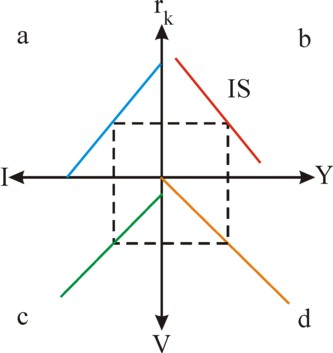Figure 1: IS-cross with Y, rk, I and V

In the formula 3 Io is the autonomous investment, and i is a constant. Incidentally, the argument of the IS/LM model does not depend in a critical manner on the precise form of the investment function2. The figure 1 illustrates the functional dependencies. She shows the national income Y, the profit rate rk, the investment I and the real demand V as a cross with graphs in each of the four quadrants. In the cross the graph in the quadrant a represents the linear investment function. The remainder of the figure 1 will be explained in the following text.

An equilibrium is formed, when the following condition is satisfied

(4)     S = I

When the formulas 2 and 3 are inserted in the formula 4, then one finds for the national income

(5)     Y = (Io + Co − i × rk) / s

and for the rate of interest rk the inverse formula

(6)     rk = (Io + Co − s × Y) / i

The formula 4 shows that now the condition for equilibrium does not prescribe a unique height of the national income. Depending on the rate of interest various values of Y(rk) are possible, where Y decreases for an increasing value of rk. This is logical, for then the investments I(rk) also decrease. The formula 6 is called the IS curve. The slope of the IS curve is -s/i, and she determines the sensitivity of Y for a changing rate of interest. According as s/i falls, and thus the curve becomes flatter, Y(rk) reacts more vehemently on changes in rk.

The IS curve can also be constructed in a graphical manner. This is done in the cross of the figure 1, resulting in the curve in the graph in the quadrant b. The method is as follows: for each value of rk the corresponding value of Y(rk) can be found by following the path a-c-d-b. On this path the graph in the quadrant c is simply the relation v=C+I from the column about the Harrod-Domar model. And the graph in the quadrant c is simply the relation Y=V, which is also copied from the mentioned column. For, in a situation of equilibrium the national income Y must equal the effective demand V of the population. Thus one finds finally the wanted value Y(rk) as the intersection of the dashed rectangle and the Y-axis in the quadrant b.

### The monetary sphere

The LM part is discussed here for the first time on the webportal. It describes how the demand and the supply of money equilibrate, and for this purpose uses the concepts, that have been developed by Keynes in his General theory. In the column about money a reference is made to the money demand due to the precaution- and speculation-motive. There it is argued, that the demand function of money can be separated in two parts:

(7)     L = Lt + Ls

Lt is the transaction part of the money demand, and results from the payments, that must be made during all kinds of transactions. According to the Verkehrsgleichung P×T = M×U, more money M is needed for the completion of the transactions T, according as T will rise. An increasing number of transactions implies, that the national income has grown. So the transaction part of the demand behaves as Lt = α×Y.

The other part Ls describes the money demand, which emerges from the speculation cashes. The investors keep liquid means in order to allow for possible investments in activities with a high capital efficiency3. According as the rate of interest rises, the investors will be less willing to keep their money liquid. The chance of a lucky shot does not compensate the lost interest. This speculative behaviour is described by the equation Ls = Lo – β × rk. After the insertion of the forms of the transaction- and speculation-demand in the formula 7 one has4

(8)     L = α × Y + Lo − β × rk

The two forms of the money demand compete for the available supply of money. When L remains unchanged, and the rate of interest rises, then Y will be pushed upwards. The money flows from the speculation cash into the transaction cash. The households will prefer to spend their money on products instead of temporarily keeping it5. Then the circulation velocity U of the money must increase, that is to say, rk influences U. The dependency of the money demand on rk is shown in the graph in the quadrant a of the cross in the figure 2. The depicted relation supposes that Y remains unchanged. The demand curves for the other Y-values are parallel to the drawn curve.

The supply function of money is given by the equation

(9)     M = Mo

Here Mo is the quantity of money, that is maintained by the Central Bank in the manner, as is described in the column about the money- and capital-markets6. The condition of equilibrium for the quantity of money is

(10)     L = M

The figure 2 shows this equilibrium relation by the graph in the quadrant c. When the formulas 8 and 9 are inserted in the formula 10, then one finds for the national income the formula

(11)     Y = (Mo − Lo + β × rk) / α

and for the rate of interest the inverse formula

(12)     rk = (α × Y − Mo + Lo) / β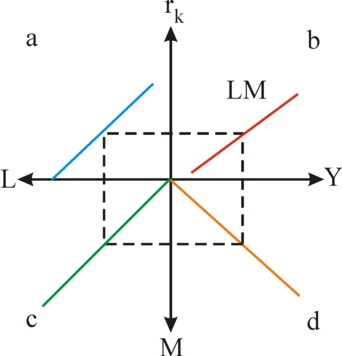Figure 2: LM-cross with Y, rk, L en M

The formula 11 is called the LM-curve. She can be constructed graphically by means of the cross in the figure 2. In the graph in the quadrant b, for each value of rk the corresponding value of Y(rk) can be found by following the path a-c-d-b. Here the graph in the quadrant d represents a variant of the Verkehrsgleichung, namely M = Y/U. This depicted relation holds for the rk, which is chosen as the starting point of the construction procedure of Y(rk). That is to say, the cobwebb is followed for a certain value of rk, so that U is also determined uniquely. Finally one finds Y(rk) as the intersection of the dotted rectangle and the Y-axis, so that the construction of this point on the LM-curve is completed. The other points are found in the same manner.

Note that the condition of equilibrium in the formula 12 can only be satisfied, as long as one has Mo > Lo. For, with rk=0 Y will still be positive. The money quantity must be larger than the maximal demand for the speculation cash. A limit also occurs for a very large rate of interest. Namely, the speculation part of the money demand equals Ls = M – Lt = Mo – α×Y. The money demand can not be negative, because then the speculators would be suppliers of money. That is not possible, because only the banks can create money. Therefore one always has Ls≥0, and so Y ≤ Mo/α.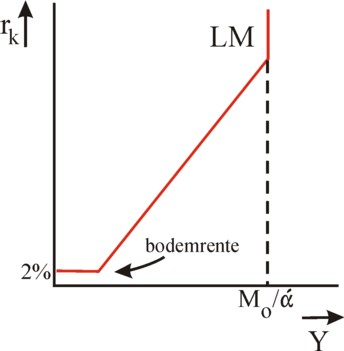Figure 3: LM curve

A national income of Mo/α implies that all speculation cashes will be empty, and the whole money quantity Mo is used for transactions. The Verkehrsgleichung shows, that then the maximal growth is reached, at least as long as the price level P does not fall or the circulation velocity U does not rise further. The circulation velocity has reached its natural maximum, because all the passive money in the cashes has been pulled out.

Note furthermore, that according to the formula 12 the interest becomes negative for low national incomes. This is an imperfection of the used function for the interest rate. A very low interest rate will actually raise the expectation with the investors, that she can only rise in the future. In this situation the investors will have an almost unlimited demand of money, because they expect to be able to invest the money in the future against a higher rate of interest. Therefore the rate of interest can commonly not fall below a margin of approximately 0% to 2%. This is called the bottom interest.

If the central bank would increase the money quantity Mo in this situation, then it will be hoarded immediately by the investors. Keynes calls this the liquidity trap. The extra money does not become available for the expansion of the number of transactions, so that the national income remains at its low level7. The figure 3 shows the LM curve, including the bottom interest and the empty speculation cashes at high rates of interest8.

### The simultaneous equilibrium

At the beginning of this column it has been stated, that the real and monetary sphere mutually interact9. Both spheres are connected by means of the rate of interest, which realizes the equilibrium condition YIS = YLM. Thanks to the formula 5 and 11 it is always possible to calculate the rate of interest for this equilibrium condition:

(13)     rk,e = ((Io + Co)/s − (Mo − Lo)/α) / (i/s + β/α)

It has already been noted, that due to the chosen form of the demand- and supply-functions the validity of the interest-formula 13 is restricted to the range of rates of interest between 0.02 and Lo/β. The national income Y(rk,e) in the state of equilibrium equals

(14)     Y = ((Io + Co )/i + (Mo − Lo)/β) / (s/i + α/β)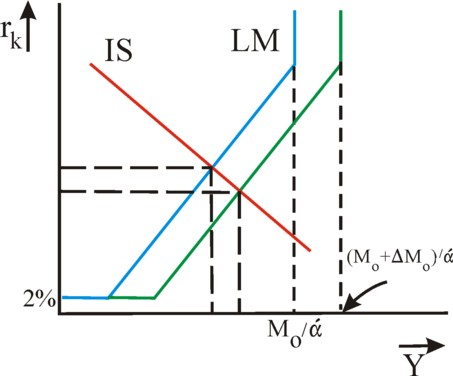Figure 4: equilibrium in the IS/ML model

The formulas 13 and 14 give insight into the way, in which the equilibrium shifts due to a change in the autonomous variables or the constant quotes. Incidentally, the dependencies can also be depicted visually by drawing the formulas 6 and 12 together in a Y - rk cross of axes. This is done in the figure 4. In particular, it becomes clear from the formula 14, that the Central Bank can increase the national income by making more money Mo available. Then in the figure 4 the LM-curve shifts downward and to the right. The income will rise, and the rate of interest will fall. In this policy the growth is probably reinforced, because the producers are incited to increase their autonomous investments Io. In that situation the rise of the investment level would result in a fairly constant rate of interest, in spite of the larger quantity of money.

When the IS/LM model is extended with the state sector, then a planned policy is possible, which uses the increase of the quantity of money as a support for expansive investments by the state. Then the state can stimulate the economy, without the risk that all the available money is hoarded. This is called an accomodating policy of the Central Bank. A detailed discussion of this theme is beyond the scope of the column. However, in the column about money it has already become apparent, that the monetarists reject the accomodation.

The term Io + Co in the formula 14 has evidently a Kaleckian-Keynesian origin, whereas in the term Mo − Lo the monetarism of the formula Y = ν × M can be recognized. The last term has an extra multiplier i/β, in comparison with the first term. If the multiplier would be much less than 1, then the post-keynesians would be right. Unfortunately it is not possible to measure its value in an empirical manner. It is actually uncertain, that for instance the LM-curve really has the shape of the figure 3. It is conceivable, that the parameters α and β themselves depend somewhat on Y. Then the LM curve can be a curved curve, such as in the figure 5, instead of a straight line. Indeed the curve is depicted in this alternative manner in many textbooks10.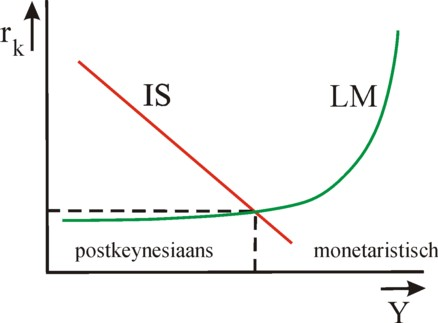Figure 5: model with curved LM curve

In the figure 5 two ranges of Y-values can be distinguished. For the lower national incomes the LM curve is almost horizontal, with as a consequence that the investments can be increased without raising the rate of interest rk. That is to say, during a shift of the IS-curve to the right rk in the intersection with the LM-curve hardly rises. Apparently, in this range of Y-values the economy behaves in a post-keynesian manner. Note also, that a (not too large) change of the money quantity M hardly influences the national income Y, because the intersection remains at almost the same place.

On the other hand, for larger national incomes the LM-curve exhibits a steep increase. Now the shift of the LM curve due to a changing money quantity M has a much more drastic effect, wherein both Y and rk must adapt. Apparently, this range of Y-values belongs to an economy with monetaristic characteristics. Now, an increase of the investments will indeed hardly yield a larger national income. The steep LM curve keeps U almost equal (but not the rate of interest!). In the paragraph about the monetary sphere it has already been explained, that here the speculation cashes are almost empty.

Apparently, the controversy between the monetarists and the post-keynesians reflects the absolute validity of each of these theories within a certain range. Between these two ranges the economy is in a state, where both the expenditures and the money quantity exert an influence. This could be called the synthesis of the two theories, but that is not entirely justified. For, the controversy concerns the question, what range is normal for the real economy11. Additional remarks can be made about the IS/LM model. For instance, the price behaviour is not considered. In a following column the model will be refined, and a judgement will be given concerning its credibility.

1. In this paragraph the explanation of A.J. Marijs en W. Hulleman in their book Macro-economie en bedrijfsomgeving (2000, Wolters-Noordhoff bv) is broadly followed. Besides, Macroeconomics (2000, Worth Publishers) by N.G. Mankiw and Macro-economie (1980, Het Spectrum) by J. Pen have been consulted extensively. (back)
2. On p.345 of Handboek economie (1978, Het Spectrum) P.A. Samuelson calls the function the curve of the marginal investment-efficiency. The formula 3 is similar to the theory, which has been developed by Keynes. According to him the producer will invest more, according as his expected capital efficiency rises further above the level of the rate of interest rk. In other words, the investments can be described by the function als I=f(r, rk). Keynes believes, that this growth of the investments is limited in a natural way. Namely, the investments will finally compel to adapt the expectations about the future, because the increased demand for means of production (department I) will push up their price. This raises the costs and reduces the expectation of profit, which were the motive for the extra investments. The formula 3 has elicited criticism form various economists:
• M. Kalecki criticizes on p.270 of Krise und Prosperität im Kapitalismus (1987, Metropolis Verlag) the argument of Keynes, because it is a static theory. According to Kalecki the investments will create additional income for the branch of the means of production (department I), and therefore this branch will cause an increased effective demand, among others for consumer goods (department II). The consumer goods can rise in price, and this may mend the expectation for profit in the department II. The rising costs of the means of production are compensated by the rising price of the consumer goods, the profit is maintained, and the prosperity can continue. Therefore on p.44 Kalecki omits the rate of interest rk in his investment function, and many post-keynesian economists have followed his example.
• I.I. Pasinetti criticizes on p.46 of Growth and income distribution (1974, Cambridge University Press) the formula 3, because she suggests a mutual dependency of I and rk. Keynes believes indeed, that the rate of interest influences the willingness to invest. However, he denies, that the investments can influence the rate of interest. In the formula 3, which is attributed to Hicks, deficit spending by the state, which raises I, would automatically cause a rising rate of interest. In this view the private investments are in danger of being pushed away by the deficit spending. However, this so-called crowding out will not occur in practice. The formula 3 describes the neoclassical picture, with the rate of interest as the stabilizing go-between, which deviates from the theory of Keynes.
(back)
3. The unearned income has a bad reputation. The group Vuile Mong en de Vieze Gasten (in Ghent) writes the poem De tango van de grondspekulant: You ask what we produce, / what makes our firm prosper? / I tell you, but hold your tongue, / we trade in goods and land! / You say that we produce nothing, / that we are merely parasites. / But dear friend, I tell you, / your reproach is unfounded! / Speculation is healthy, / the sale of green and land. / When tomorrow nothing remains / we have the interest. / You also ask me to illustrate / that we manipulate the market. / I say it loudly and plainly: / that it done by the Farmers' Association. / You believe that we do not honour the price / but allow me to protest. / We sit here on our ass / and the price of land rises. (back)
4. Pasinetti states that the equation is also faulty, because she supposes a mutual dependency between Y and the demand for money. For, the theory of Keynes assumes that the dependency is one-sided. The demand for money determines the rate of interest; the rate of interest determines the investments; the investments determine the national income; that is to say, L → rk → I → Y. However, the reverse functional relation, L=f(Y) such as in the formula 8, does not hold. Samuelson also assumes in chapter 18 of Handboek economie the one-sided causal relation, but starts the chain from the supply side, that is to say with M instead of L. The central bank uses M to steer the granting of credits. He calls the formula 8 the curve of the liquidity preference. And according to p.166 of Volkswirtschaftslehre (2003, Oldenbourg Wissenschaftsverlag GmbH) the Kaleckian-Keynesian theory has a market hierarchy, which starts with the capital market. This market affects the market of investment goods, and this in turn affects the labour market. (back)
5. The speculation part consists probably mainly of term- and savings-deposits with a minimal compensation in interest. (back)
6. According to Mankiw in the already mentioned Macroeconomics M is less relevant than the real supply of money M/P, where P is the price level, just like before. This opinion induces Mankiw to base his explanation on the number of transactions T, instead of on the national income, such as is done in this column. According to Mankiw the condition of equilibrium is L’=M/P, where L’ is the demand for a real money sum. In other words, the demand of the households is a real quantity, and not a nominal one. Therefore it must be remembered that for convenience the presented argument (which agrees with Macro-economie en bedrijfsomgeving by Marijs and Hulleman) has excluded the possible changes in the price level. (back)
7. E. Feess-Dörr shows on p.131 of Die Redundanz der Mehrwerttheorie (1989, Metropolis Verlag), that Marx expects in volume 3 of Das Kapital a rising rate of interest during the recession, already before Keynes did. When Y decreases in the recession, then the rate of interest does not fall according to the formula 12, because the producers desperately need credits. However, the capital owners have such low expectations with regard to the profitability of the enterprises, that they only want to loan money against a high interest. This is apparently a somewhat different presentation of the liquidity trap. (back)
8. The behaviour of the LM curve at its ends shows, that the fundamental monetaristic assumption Y = ν × M has at most a limited validity. The formula 8 also shows, that the speculation part of the money demand does not directly relate to Y. (back)
9. Also this equilibirum causes a social controversy. The Flemish bard Walter de Buck, an inhabitant of Ghent as well, writes in the poem De wereld van vandaag: Ministers, presidents / of the rich world / play with their money / the last judgement or more. / By lying and deceit, by weapons and violence, / and by soothing the people / they gain even more money. / The worker has fought / for liberty and right / and he has suffered a lot / due to priests and judges. / But what did he acquire / besides a fridge and TV? / Everything that he combatted, / now he joins it. / Alas! Old socialists, / don't you still see clearly? / A bunch of egoists / endanger your cause. Your columnist supposes that this text is ment to be satire, just like the one of Vuile Mong. However, it is questionable that all fans of De Buck will understand the humour. The controversy is formulated even more striking in a citation from Uit het dagboek van een student in the almanac of the Katholieke Studenten Vereniging Sanctus Laurentius (p.327): I nestled near the fireside. The flames could only exist due to the destruction. It is strange that such a fire poses this problem. Neither essence, nor existence are the basis of being, the first principle of something. Yet only together do they form a harmony. The problem of man is merely, that he always tends to undermine himself, to ignore his own being, as it were. In the end man has simply the task to realize himself, By his behaviour he continually creates and destroys himself. (back)
10. This is among others the case in the mentioned Macroeconomics. (back)
11. For instance, on p.362 of Handboek economie it is stated: “There are few modern economists, who think that it is possible that a modern economy can enter such an extreme classical (monetaristic) range". The post-keynesian range was entered during the Great Depression of the thirties. The situation deteriorated further, because the producers were unwilling to invest, so that the IS curve had become almost vertical. (back)